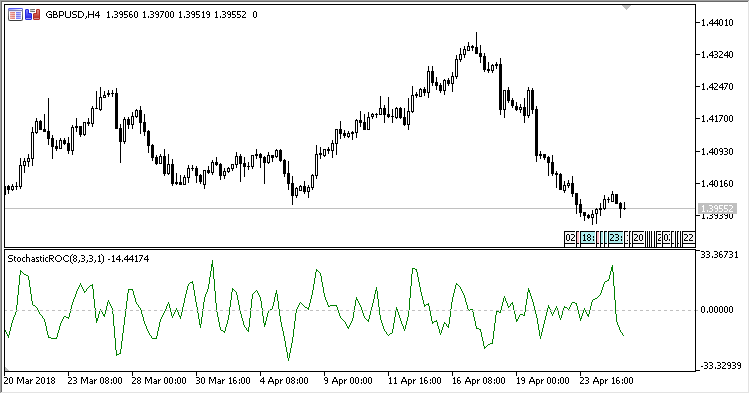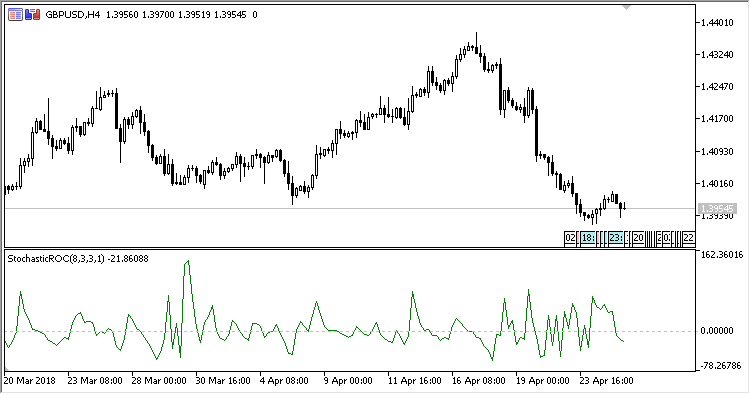# Stochastic_ROC – indicator for MetaTrader 5

• A+
category：MT5 INDICATORS

Price Rate-of-Change (ROC) indicator based on stochastic.

It has eight input parameters:

• Stochastic K period - the period of calculating the stochastic %K line;
• Stochastic D period - the period of calculating the stochastic %D line;
• Stochastic Slowing - the period of calculating the stochastic Slowing line;
• Stochastic MA Method - stochastic calculation method;
• Stochastic Price field - stochastic calculation prices;
• Stochastic line - stochastic line used for calculating the indicator:
• Main - main line;
• Signal - signal line.
• ROC period - the period of calculating the ROC;
• Calculation type - type of calculations:
• Absolute - absolute values;
• Relative - relative values.

The ROC indicator is not required for calculations, since it is calculated by the indicator itself.

Calculations:

```If Type = Absolute Stochastic, then ROC = ((Stochastic(Now) - Stochastic(Prev)),
If Type = Relative Stochastic, then ROC = ((Stochastic(Now) - Stochastic(Prev)) / Stochastic(Prev))*100
```

ROC:

```ROC = ((CLOSE (i) - CLOSE (i - n)) / CLOSE (i - n)) * 100
CLOSE (i) - the price of closing the current bar;
CLOSE (i - n) - the close price n bars ago;
```Fig.1. Stochastic ROC AbsoluteFig.2. Stochastic ROC Relative